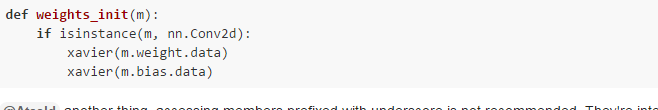# Weight initilzation

A less Lua way of doing that would be to check if some module is an instance of a class. This is the recommended way:

``````def weights_init(m):
if isinstance(m, nn.Conv2d):
xavier(m.weight.data)
xavier(m.bias.data)
``````

@Atcold another thing, accessing members prefixed with underscore is not recommended. They’re internal and subject to change without notice. If you want to get a iterator over modules use `.modules()` (searches recusrively) or `.children()` (only one level).

29 Likes

Thanks guys.
I am trying to apply weight initialization to a fully connected network (nn.Linear). I need, however, fan_out and fan_in of this layer. By fan_out and fan_in I mean number of output neurons and input neurons, respectively. How can I access them?

@Hamid, you can check the size of the `weight` matrix.

``````size = m.weight.size() # returns a tuple
fan_out = size # number of rows
fan_in = size # number of columns
``````

@apaszke, thanks for the heads-up! I’m still new to the Python world…

Edit: apply @apaszke fix.

3 Likes

A small note - `.size()` is also defined on Variables, so no need to unpack the data. `m.weight.size()` will work too.

@Hamid, are you trying to ask something? I am not sure I understand.
Could you also please format your code with three backticks and the word `python`, so that I can read what you posted?

1 Like

@Atcold, by checking if isinstance(m, nn.Linear) it would apply to linear module, correct?
If I call the weight initialization, it would be applied to all layers?
I have a residual module each having 2 linear layers. Then several of these modules.

my code:

``````def weight_init(m):
if isinstance(m, nn.Linear):
size = m.weight.size()
fan_out = size # number of rows
fan_in = size # number of columns
variance = np.sqrt(2.0/(fan_in + fan_out))
m.weight.data.normal_(0.0, variance)

class Residual(nn.Module):
def __init__(self,dropout, shape, negative_slope, BNflag = False):
super(Residual, self).__init__()
self.dropout = dropout
self.linear1 = nn.Linear(shape,shape)
self.linear2 = nn.Linear(shape,shape)
self.dropout = nn.Dropout(self.dropout)
self.BNflag = BNflag
self.batch_normlization = nn.BatchNorm1d(shape)
self.leakyRelu = nn.LeakyReLU(negative_slope = negative_slope , inplace=False)

def forward(self, X):
x = X
if self.BNFlag:
x = self.batch_normlization(x)
x = self.leakyRelu(x)
x = self.dropout(x)
x = self.linear1(x)
if self.BNFlag:
x = self.batch_normlization(x)
x = self.leakyRelu(x)
x = self.dropout(x)
x = self.linear2(x)
return x

class FullyCN(nn.Module):
def __init__(self, args):
super(FullyCN, self).__init__()
self.numlayers = arg.sm-num-hidden-layers
self.learning-rate= args.sm-learning-rate
self.dropout = arg.sm-dropout-prob
self.BNflag = args.sm-bn
self.shape = [args.sm-input-size,args.sm-num-hidden-units]
self.res =  Residual(self.dropout,self.shape,args.sm_act_param,self.self.BNflag)
self.res(weight_init)
self.res-outpus = []

def forward(self,X):
self.res-outpus.append(self.res(X))
for i in range(self.numlayers):
self.res-outpus.append(self.res(self.res-outpus[-1]))
return self.res-outpus[-1]``````
2 Likes

Correct.

Yup. All linear layers.

Sure, it will apply the normalisation to each `Module` that belongs to the class `nn.Linear`.

But I have called weight_init once for the class while I call linear layers in a for loop (i.e., there are multiple sets of variables).

``````net = Residual() # generate an instance network from the Net class
net.apply(weights_init) # apply weight init
``````

I’m not too sure what you’re doing with `FullyCN`

1 Like

The `apply` function will search recursively for all the modules inside your network, and will call the function on each of them. So all `Linear` layers you have in your model will be initialized using this one call.

9 Likes

@Atcold, in FullyCN I use several residual modules. In another piece of code, I pass data to FullyCN which returns corresponding output via its forward function.

How can we simply revise the parameters of a pretrained model in torchvision? For example, how can we revise each stride parameter of models.resnet101 in each layer? Can we set sth. just like model.conv1.stride=1?
What I can see is to write down a new model, revise the weights of the pretrained model, and copy it to the new model by net.apply(weights_init). Is there any simpler way?

Why would you need to “revise” (edit, change?) a convolutional stride of a pre-trained model?
I’m not sure I understand what you are after…

I’d recommend recreating the model - this is going to work for sure. You probably could monkey-patch the strides with a new tuple (try seeing what’s the type of this attribute in the loaded model). However keep in mind that the model was trained with different parameters, it might now work too well after that change.

Change some parameters of a pretrained model, just like ‘Going Deeper on the Tiny Imagenet Challenge’ has done. The author changed the input image size. Consequently, the filter size has to be changed. I have to do similar things. Therefore, I asked the problems. O(∩_∩)O

Thank @apaszke for detailed explanation, and @Atcold for reminder.

Is this way include paramters in Batch normalization?